Foxtable(狐表)用户栏目专家坐堂 → 如何读取文本数据写入数据表？

共有364人关注过本帖树形打印复制链接

主题：如何读取文本数据写入数据表？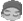cnsjroom
1楼 | 信息 | 搜索 | 邮箱 | 主页 | UC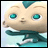加好友发短信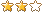下载信息  [文件大小：   下载次数： ]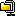点击浏览该文件:文本数据.zip

Output.Show(s.split("|").Length-1)
If s.split("|").Length-1 = 21 Then
s.Insert(21,chr(13) & chr(10))
End If
Dim Values1() As String = s.split(chr(13))
Output.Show(Values1(0))

Dim Multi As String = "账号|户名|交易日期|主机日期|交易时间|交易码|交易名称|借贷标记|交易金额|账户余额|营业机构|账务机构|交易柜员|授权柜员|柜员流水|现转标志|摘要代码|对方账号|对方名称|对方行号|对方行名"
Dim Values() As String
Values = Multi.split("|")
Dim dtb As New DataTableBuilder("统计")
For Index As Integer = 0 To Values.Length - 1
Output.Show(Values(Index))
Next
dtb.Build()
[此贴子已经被作者于2021/11/25 18:03:22编辑过]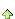cnsjroom
2楼 | 信息 | 搜索 | 邮箱 | 主页 | UC加好友发短信老师  参照过这个帮助   得到的结果和文本是一样的 还得麻烦老师根据我这个银行导出的text文本来指导下   文档已放入楼上的文件中cnsjroom
3楼 | 信息 | 搜索 | 邮箱 | 主页 | UC加好友发短信老师我参照操作了下   运行效果如图（红色部分因为没有|符号  所以没有正确拆分  老师有没有办法呢   我试过换行符  回车符 都没有行）如果正确拆分之后，又该怎么写入对应的列名下的值呢？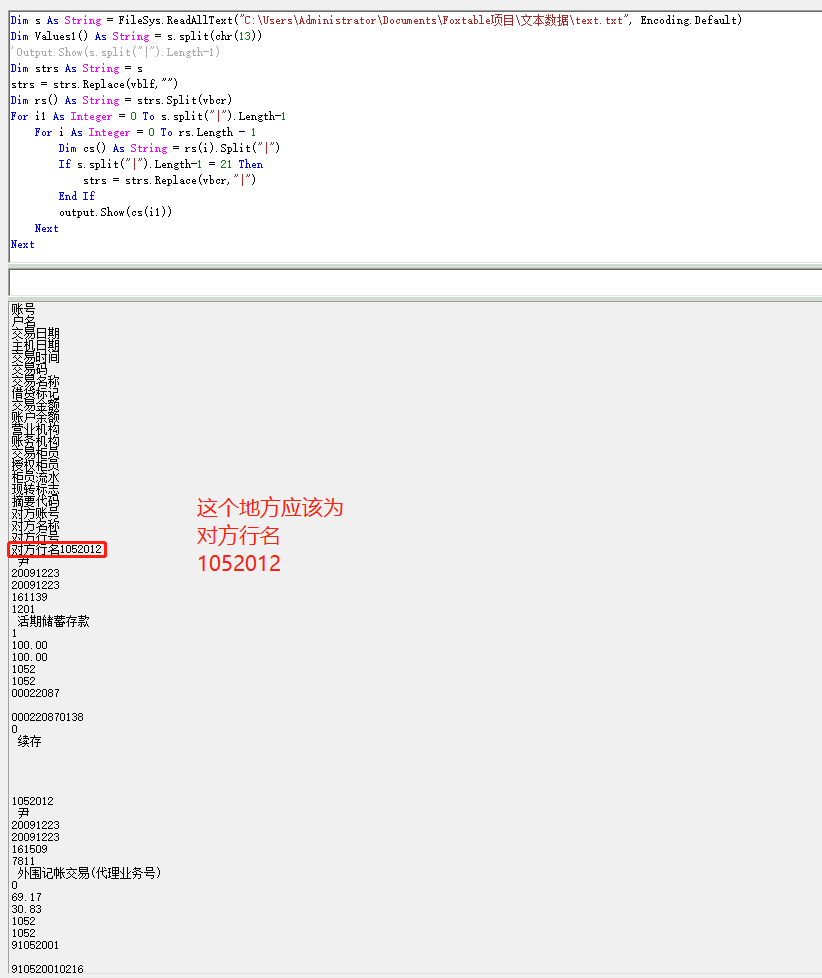当前代码如下：Dim s As String = FileSys.ReadAllText("C:\Users\Administrator\Documents\Foxtable项目\文本数据\text.txt", Encoding.Default)Dim Values1() As String = s.split(chr(13))'Output.Show(s.split("|").Length-1)Dim strs As String = sstrs = strs.Replace(vblf,"")Dim rs() As String = strs.Split(vbcr)For i1 As Integer = 0 To s.split("|").Length-1        For i As Integer = 0 To rs.Length - 1        Dim cs() As String = rs(i).Split("|")        If s.split("|").Length-1 = 21 Then            strs = strs.Replace(vbcr,"|")        End If        output.Show(cs(i1))    Next   Next文件内容如下：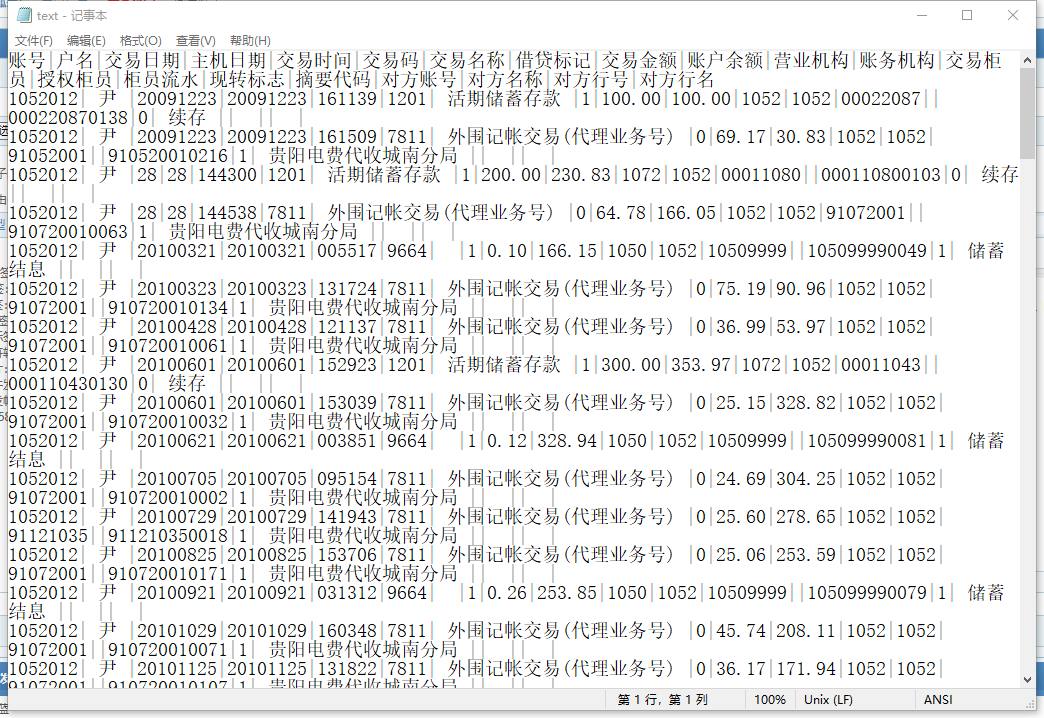列名和临时表生成代码：Dim Multi As String = "账号|户名|交易日期|主机日期|交易时间|交易码|交易名称|借贷标记|交易金额|账户余额|营业机构|账务机构|交易柜员|授权柜员|柜员流水|现转标志|摘要代码|对方账号|对方名称|对方行号|对方行名"Dim Values() As StringValues = Multi.split("|")Dim dtb As New DataTableBuilder("统计")For Index As Integer = 0 To Values.Length - 1    Output.Show(Values(Index))    dtb.AddDef(Values(Index), Gettype(String), 32)Nextdtb.Build() [此贴子已经被作者于2021/11/26 10:10:32编辑过]cnsjroom
4楼 | 信息 | 搜索 | 邮箱 | 主页 | UC加好友发短信谢谢老师  精简的代码   获取得到数据之后  当前采用了临时表代码生成临时表怎么匹配拆分的值到对应的列名下呢？Dim s As String = FileSys.ReadAllText("C:\Users\Administrator\Documents\Foxtable项目\文本数据\text.txt", Encoding.Default)Dim Values1() As String = s.Replace(vbcr,"").split(vblf)For i As Integer = 0 To Values1.length - 1    Dim cs() As String = Values1(i).split("|")    For i1 As Integer = 0 To cs.Length-1        output.Show(cs(i1))    NextNext列名和临时表生成代码：Dim Multi As String = "账号|户名|交易日期|主机日期|交易时间|交易码|交易名称|借贷标记|交易金额|账户余额|营业机构|账务机构|交易柜员|授权柜员|柜员流水|现转标志|摘要代码|对方账号|对方名称|对方行号|对方行名"Dim Values() As StringValues = Multi.split("|")Dim dtb As New DataTableBuilder("统计")For Index As Integer = 0 To Values.Length - 1    Output.Show(Values(Index))    dtb.AddDef(Values(Index), Gettype(String), 32)Nextdtb.Build() [此贴子已经被作者于2021/11/26 10:22:45编辑过]cnsjroom
5楼 | 信息 | 搜索 | 邮箱 | 主页 | UC加好友发短信不好意思   老师我按照帮助教程还是没有得到要领当前代码如下：Dim Multi As String = "账号|户名|交易日期|主机日期|交易时间|交易码|交易名称|借贷标记|交易金额|账户余额|营业机构|账务机构|交易柜员|授权柜员|柜员流水|现转标志|摘要代码|对方账号|对方名称|对方行号|对方行名"Dim Values() As StringValues = Multi.split("|")Dim dtb As New DataTableBuilder("统计")For Index As Integer = 0 To Values.Length - 1    Output.Show(Values(Index))    dtb.AddDef(Values(Index), Gettype(String), 32)Nextdtb.Build()MainTable=Tables("统计")Dim s As String = FileSys.ReadAllText("C:\Users\Administrator\Documents\Foxtable项目\文本数据\text.txt", Encoding.Default)Dim Values1() As String = s.Replace(vbcr,"").split(vblf)For i As Integer = 0 To Values1.length - 1    Dim cs() As String = Values1(i).split("|")    For i1 As Integer = 0 To cs.Length-1        'output.Show(cs(i1))        Dim cs1() As String = cs(i1).Split("|")        output.Show(cs1(0))        If cs1.Length = 21 Then            'output.Show(cs(i1))                        For Each c As DataCol In DataTables("统计").DataCols                                'output.Show(c.name)                                               Dim dr As DataRow = DataTables("统计").Addnew()                dr(c.name) = cs1(0)                'dr("第二列") = cs(1)                'dr("第三列") = cs(2)                'dr("第四列") = cs(3)                            Next        End If    NextNextcnsjroom
6楼 | 信息 | 搜索 | 邮箱 | 主页 | UC加好友发短信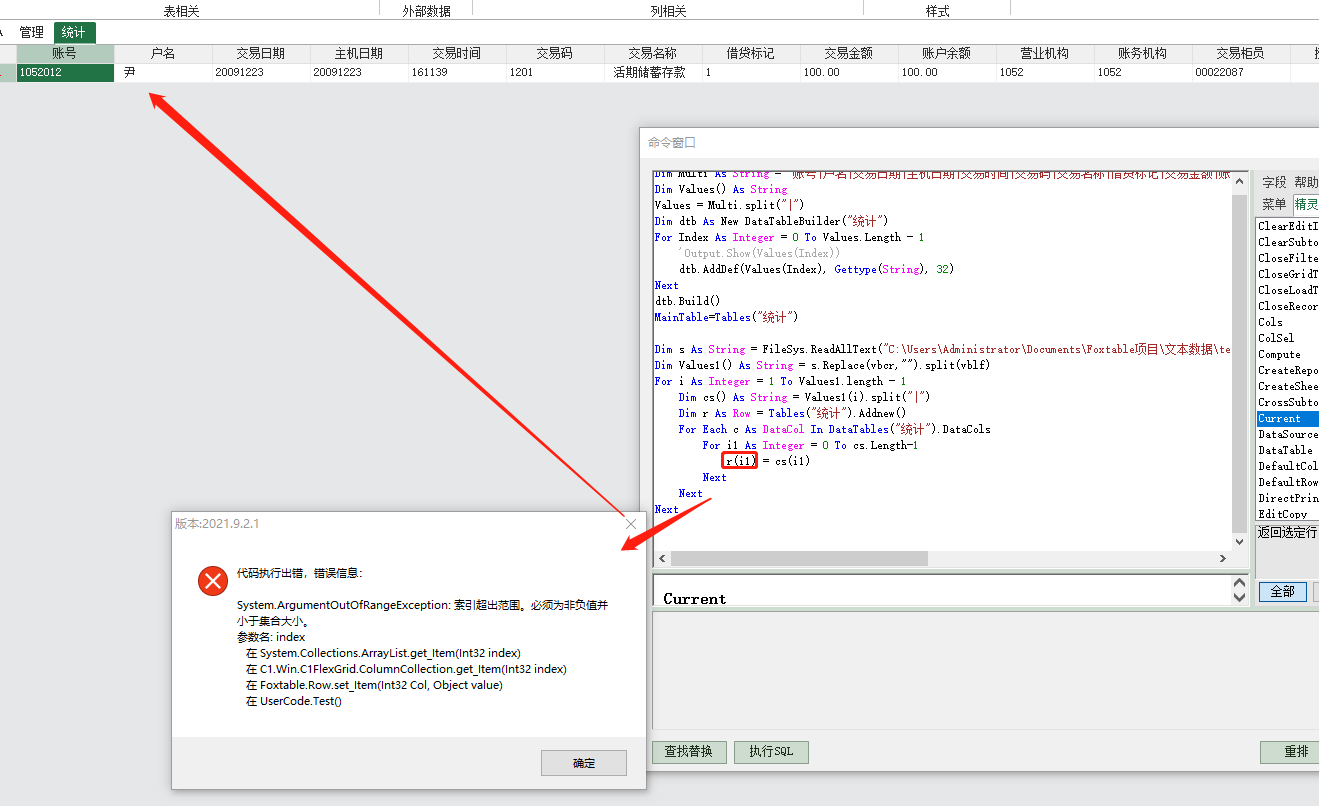老师运行提示如图错误cnsjroom
7楼 | 信息 | 搜索 | 邮箱 | 主页 | UC加好友发短信Dim s As String = FileSys.ReadAllText("C:\Users\Administrator\Documents\Foxtable项目\文本数据\text.txt", Encoding.Default)Dim Values1() As String = s.Replace(vbcr,"").split(vblf)For i As Integer = 1 To Values1.length - 1    Dim cs() As String = Values1(i).split("|")    Dim r As Row = Tables("统计").Addnew()    'For Each c As DataCol In DataTables("统计").DataCols    output.show(cs.Length-1)    For i1 As Integer = 0 To cs.Length-1        If cs.Length-1 = 1 Then            r(i1) = cs(i1)        End If    Next    'NextNext数据行对了 但是是空行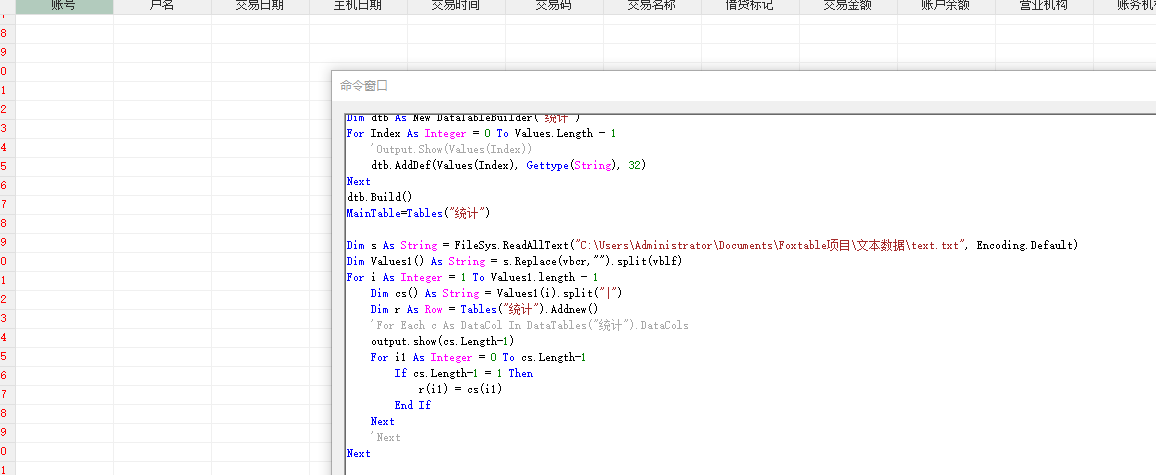cnsjroom
8楼 | 信息 | 搜索 | 邮箱 | 主页 | UC加好友发短信麻烦老师帮忙看看   为什么还是不行呢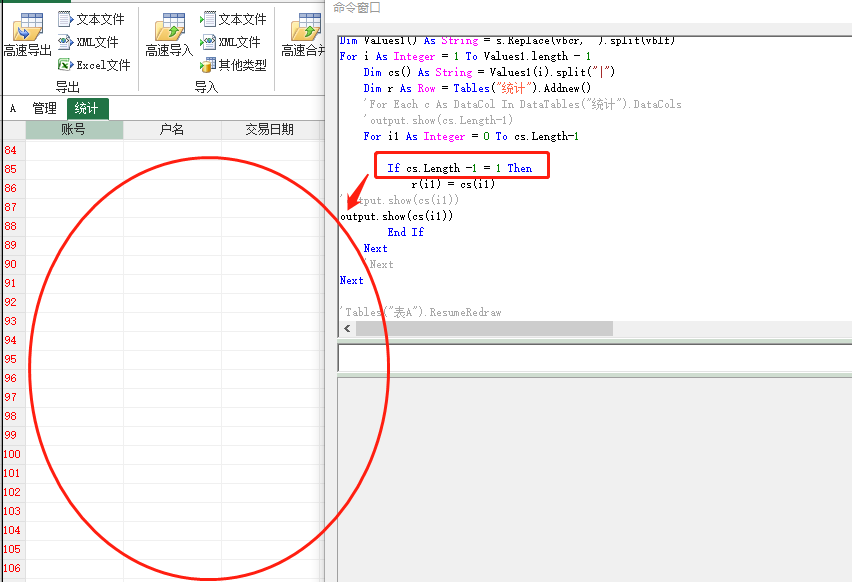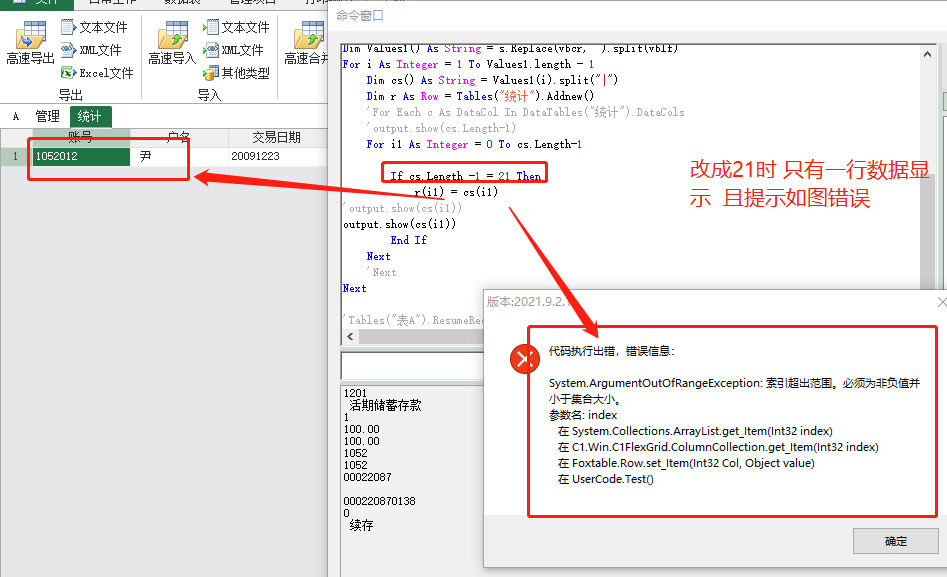[此贴子已经被作者于2021/11/26 19:39:18编辑过]